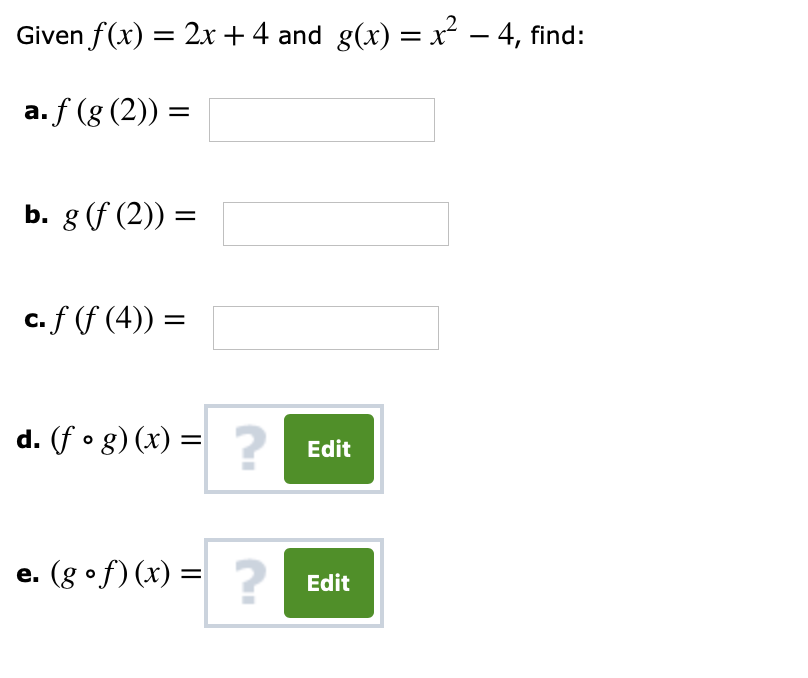Given f(x) = 2x + 4 and g(x) = x2-4, find:aJUg (2)) =b, g (f (2)) =c.f(f(4)) =d.(fog) (x) =2EditEdit

Questionhelp_outlineImage TranscriptioncloseGiven f(x) = 2x + 4 and g(x) = x2-4, find: aJUg (2)) = b, g (f (2)) = c.f(f(4)) = d.(fog) (x) = 2 Edit Edit fullscreen
Step 1

Calculation:

The given functions are f(x) = 2x +4 and g(x) = x2 - 4.

a) Calculate the value of the composition function “f(g(x))” as follows.

Step 2

Obtain the value of the composition function “f(g(x))” at the point x = 2 as follows.

Step 3

b) Calculate the value of the composition function...

Want to see the full answer?

See Solution

Want to see this answer and more?

Our solutions are written by experts, many with advanced degrees, and available 24/7

See Solution
Tagged in

Other